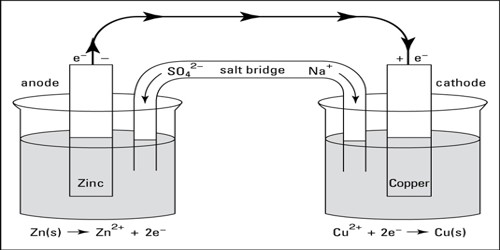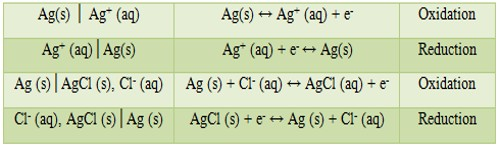Chemistry

# Electrochemical Cells: Notations and Sign ConventionElectrochemical Cells: Notations and Sign Convention

An electrochemical cell may be prepared by combining any two half-cells. Such cells and half-cells are conveniently described by an abbreviated notation together with appropriate conventions so that the convention will correspond to the chemical reaction taking place in the cell. Moreover, the conventions used for the cells must be compatible with other thermodynamic conventions. The following notations are used by convention for describing cells and half-cells:

(1) The half-cells written in the sequence electrode│electrolyte represents oxidation and if the sequence is electrolyte│electrode, it means reduction. Examples are:Note that gas electrodes or oxidation-reduction electrodes have an inert metal (platinum) as the electron carrying medium.

(2) A complete cell is written such that the left hand side represents the oxidation reaction half-cell (anode) and the right hand side represents the reduction half-reaction half-cell (cathode) cell as shown below:

Zn (s)│ZnSO4 (aq)│CuSO4 (aq)│Cu (s)

The vertical line in the middle means that the two solutions are in direct contact. If, however, contact between the two solutions is made through a salt bridge two vertical lines are placed between the two electrolytes as shown below:

Zn (s)│ZnSO4 (aq)││CuSO4 (aq)│Cu (s)

These are also written simply as-

Zn (s)│Zn2+ (aq)││Cu2+ (aq)│Cu (s)

(3) The sign of the electrodes are opposite to those used for electrolytic cells:

• Electrochemical cells: Anode is negative and Cathode is positive.
• Electrolytic cells: Anode is positive and Cathode is negative.

(4) The electrode where electron is released, i.e., oxidation takes place is written on the left side and is the anode and the cathode is written on the right side. An e.m.f. will be called positive if there is a tendency for electrons to be driven through the external circuit from left to right. If the cell is written such that reduction is taking place at the left hand electrode, the e.m.f. of the cell will be negative.

(5) For calculating the cell potential always reduction potential is used along with the relation.

(6) If the e.m.f of the cell has a positive value the cell reaction is spontaneous, i.e., if the cell works in such a way that electron is released at the left electrode and is driven through the external circuit from left to right the cell reaction will be spontaneous.